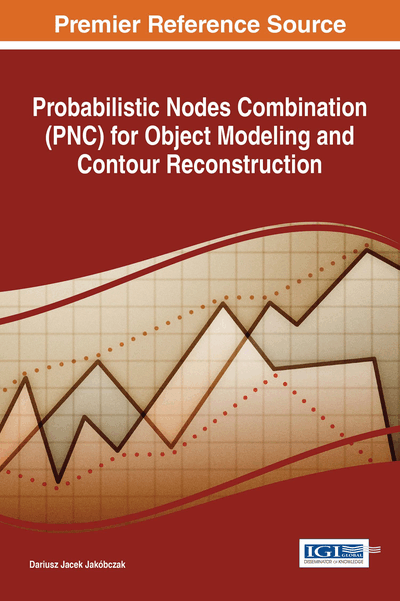# Applications of PNC in Numerical Methods

DOI: 10.4018/978-1-5225-2531-8.ch007
Available
\$37.50
No Current Special Offers

## Abstract

Nodes are treated as characteristic points of data for modeling and analyzing. The model of data can be built by choice of probability distribution function and nodes combination. Two-dimensional object is extrapolated and interpolated via nodes combination and different functions as discrete or continuous probability distribution functions: polynomial, sine, cosine, tangent, cotangent, logarithm, exponent, arc sin, arc cos, arc tan, arc cot or power function. Curve interpolation represents one of the most important problems in mathematics and computer science: how to model the curve via discrete set of two-dimensional points? Also the matter of shape representation (as closed curve - contour) and curve parameterization is still opened. For example pattern recognition, signature verification or handwriting identification problems are based on curve modeling via the choice of key points. So interpolation is not only a pure mathematical problem but important task in computer vision and artificial intelligence.
Chapter Preview
Top

## Introduction

Numerical modeling is still developing branch of the computer science: operational research (for example probabilistic model-based prognosis) (Lorton, Fouladirad & Grall, 2013), decision making techniques and probabilistic modeling (Pergler & Freeman, 2008), artificial intelligence and machine learning. There are used different aspects of probabilistic methods: stochastic processes and stochastic model-based techniques, Markov processes (Cocozza-Thivent, Eymard, Mercier & Roussignol, 2006), Poisson processes, Gamma processes, a Monte Carlo method, Bayes rule, conditional probability and many probability distributions. In this chapter the goal of probability distribution function is to describe the position of unknown points between given interpolation nodes. Two-dimensional curve (opened or closed) is used to represent the data points. So problem statement of this chapter is: how to reconstruct (interpolate) missing points of 2D curve having the set of interpolation nodes (key points) and using the information about probabilistic distribution of unknown points. For example the simplest basic distribution leads to the easiest interpolation – linear interpolation. Apart from probability distribution, additionally there is the second factor of proposed interpolation method: nodes combination. The simplest nodes combination is zero. Thus proposed curve modeling is based on two agents: probability distribution and nodes combination. Curve interpolation (Collins, 2003) represents one of the most important problems in mathematics and computer science: how to model the curve (Chapra, 2012) via discrete set of two-dimensional points (Ralston & Rabinowitz, 2001)? Also the matter of shape representation (as closed curve-contour) and curve parameterization is still opened (Zhang & Lu, 2004). For example pattern recognition, signature verification or handwriting identification problems are based on curve modeling via the choice of key points. So interpolation is not only a pure mathematical problem but important task in computer vision and artificial intelligence. The chapter wants to approach a problem of curve modeling by characteristic points. Proposed method relies on nodes combination and functional modeling of curve points situated between the basic set of key points. The functions that are used in calculations represent whole family of elementary functions with inverse functions: polynomials, trigonometric, cyclometric, logarithmic, exponential and power function. These functions are treated as probability distribution functions in the range [0;1]. Significant problem in machine vision and computer vision is that of appropriate 2D shape representation and reconstruction. Classical discussion about shape representation is based on the problem: contour versus skeleton. This monograph is voting for contour which forms boundary of the object. Contour of the object, represented by successive contour points, consists of information which allows us to describe many important features of the object as shape coefficients. 2D curve modeling and generation is a basic subject in many branches of industry and computer science, for example in the cad/cam software. The representation of shape can have a great impact on the accuracy and effectiveness of object recognition. In the literature, shape has been represented by many options including curves, graph-based algorithms and medial axis to enable shape-based object recognition. Digital 2D curve (open or closed) can be represented by chain code (Freeman’s code). Chain code depends on selection of the started point and transformations of the object. So Freeman’s code is one of the method how to describe and to find contour of the object. Analog (continuous) version of Freeman’s code is the curve α-s. Another contour representation and reconstruction is based on Fourier coefficients calculated in discrete Fourier transformation (DFT). These coefficients are used to fix similarity of the contours with different sizes or directions. If we assume that contour is built from segments of a line and fragments of circles or ellipses, hough transformation is applied to detect contour lines. Also geometrical moments of the object are used during the process of object shape representation. Contour is also applied in shape decomposition. Many branches of medicine, industry and manufacturing are looking for methods connected with geometry of the contour. Why and when should we use MHR and PNC methods? Interpolation methods and curve fitting represent so huge problem that each individual interpolation is exceptional and requires specific solutions. PNC method is such a novel tool with its all pros and cons. The user has to decide which interpolation method is the best in a single situation. The choice is yours if you have any choice. Presented method is such a new possibility for curve fitting and interpolation when specific data (for example handwritten symbol or character) starts up with no rules for polynomial interpolation. This book consists of two generalizations: generalization of previous MHR method with various nodes combinations and generalization of linear interpolation with different (no basic) probability distribution functions and nodes combinations.

## Complete Chapter List

Search this Book:
Reset